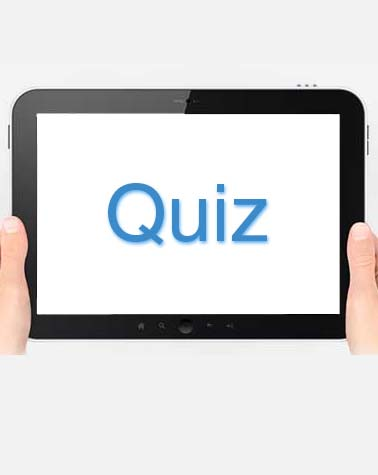# AML Quiz 4 Section B

10 Questions | Total Attempts: 67SettingsCreate your own Quiz.

• 1.
For the given set of points which of the following lines is most suitable to be the decision boundary?
• A.

Option 1

• B.

Option 2

• C.

Option 3

• D.

Option 4

• 2.
Which of the following is/are true about the Perceptron classifier? (Choose multiple option)
• A.

It can learn a OR function

• B.

It can learn a AND function

• C.

The obtained separating hyperplane depends on the order in which the points are presented in the training process.

• D.

For a linearly separable problem, there exists some initialization of the weights which might lead to non-convergent cases.

• 3.
Suppose you run K-means clustering algorithm on a given dataset. What are the factors on which the final clusters depend on ? I. The value of K II. The initial cluster seeds chosen III. The distance function used.
• A.

I only

• B.

II only

• C.

I & II only

• D.

I,II and III

• 4.
After training an SVM, we can discard all examples which do not support vectors and can still classify new examples?
• A.

True

• B.

False

• 5.
If g(z) is the sigmoid function, then its derivative with respect to z may be written in term of g(z) as
• A.

G(z)(1-g(z))

• B.

G(z)(1+g(z))

• C.

-g(z)(1+g(z))

• D.

G(z)(g(z)-1)

• 6.
The back-propagation learning algorithm applied to a two layer neural network
• A.

Always finds the globally optimal solution

• B.

Finds a locally optimal solution which may be globally optimal.

• C.

Never finds the globally optimal solution.

• D.

Finds a locally optimal solution which is never globally optimal

• 7.
Which of the following is true?
• A.

In batch gradient descent we update the weights and biases of the neural network after forward pass over each training example.

• B.

In batch gradient descent we update the weights and biases of our neural network after forward pass over all the training examples.

• C.

Each step of stochastic gradient descent takes more time than each step of batch gradient descent.

• D.

None of these three options is correct

• 8.
In a neural network, which one of the following techniques is NOT useful to reduce overfitting?
• A.

Dropout

• B.

Regularization

• C.

Batch normalizatioh

• D.

• 9.
For an image recognition problem (such as recognizing a cat in a photo), which architecture of neural network has been found to be better suited for the tasks
• A.

Multi layer perceptron

• B.

Recurrent neural network

• C.

Convolutional neural network

• D.

Perceptron

• 10.
The Bayes Optimal Classifier
• A.

Is an ensemble of some selected hypotheses in the hypothesis space

• B.

Is an ensemble of all the hypotheses in the hypothesis space

• C.

Is the hypothesis that gives best result on test instances

• D.

None of the aboveBack to top